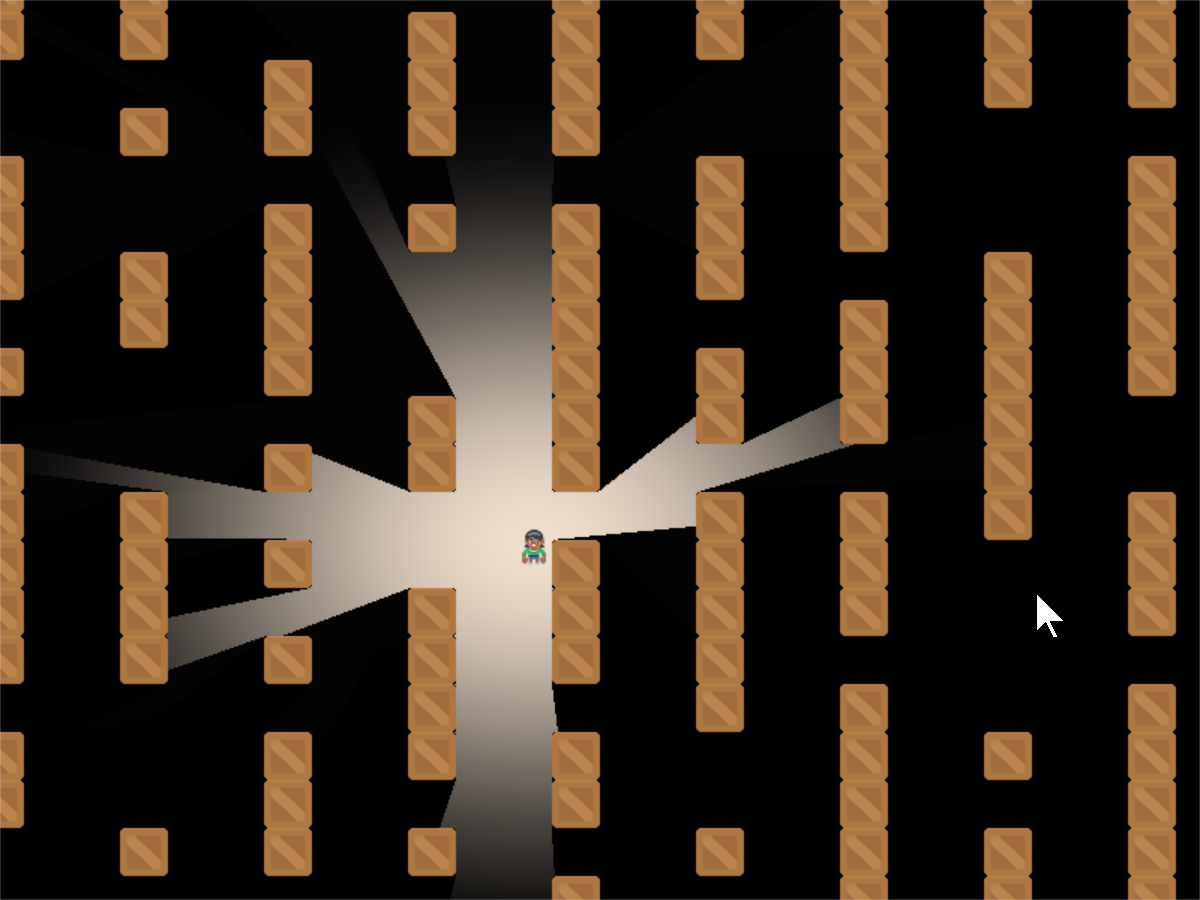A common effect for many games is ray-casting. Having the user only be able to see what is directly in their line-of-sight.

This can be done quickly using shaders. These are small programs that run on the graphics card. They can take advantage of the Graphics Processing Unit. The GPU has a lot of mini-CPUs dedicated to processing graphics much faster than your main computer’s CPU can.

## Starting Program

Before we start adding shadows, we need a good starting program. Let’s create some crates to block our vision, some bombs to hide in them, and a player character:The listing for this starting program is available at Ray-Casting Starting File.

Now, let’s create a shader. We can program shaders using Arcade’s `Shadertoy` class.

We’ll modify our prior program to import the Shadertoy class:

```from arcade.experimental import Shadertoy
```

Next, we’ll need some shader-related variables. In addition to a variable to hold the shader, we are also going to need to keep track of a couple frame buffer objects (FBOs). You can store image data in an FBO and send it to the shader program. An FBO is held on the graphics card. Manipulating an FBO there is much faster than working with one in loaded into main memory.

Shadertoy has four built-in channels that our shader programs can work with. Channels can be mapped to FBOs. This allows us to pass image data to our shader program for it to process. The four channels are numbered 0 to 3.

We’ll be using two channels to cast shadows. We will use the `channel0` variable to hold our barriers that can cast shadows. We will use the `channel1` variable to hold the ground, bombs, or anything we want to be hidden by shadows.

```    def __init__(self, width, height, title):
super().__init__(width, height, title, resizable=True)

# The shader toy and 'channels' we'll be using
self.channel0 = None
self.channel1 = None

# Sprites and sprite lists
self.player_sprite = None
self.physics_engine = None

self.generate_sprites()
```

These are just empty place-holders. We’ll load our shader and create FBOs to hold the image data we send the shader in a `load_shader` method: This code creates the shader and the FBOs:

Create the shader, and the FBOs
```    def load_shader(self):
# Where is the shader file? Must be specified as a path.

# Size of the window
window_size = self.get_size()

# Create the channels 0 and 1 frame buffers.
# Make the buffer the size of the window, with 4 channels (RGBA)
)
)

# Assign the frame buffers to the channels
```

As you’ll note, the method loads a “glsl” program from another file. Our ray-casting program will be made of two files. One file will hold our Python program, and one file will hold our Shader program. Shader programs are written in a language called OpenGL Shading Language (GLSL). This language’s syntax is similar to C, Java, or C#.

Our first shader will be straight-forward. It will just take input from channel 0 and copy it to the output.

GLSL Program for Step 1
```void mainImage( out vec4 fragColor, in vec2 fragCoord )
{
vec2 normalizedFragCoord = fragCoord/iResolution.xy;
fragColor = texture(iChannel0, normalizedCoord);
}
```

How does this shader work? For each point in our output, this `mainImage` function runs and calculates our output color. For a window that is 800x600 pixels, this function runs 480,000 times for each frame. Modern GPUs can have anywhere between 500-5,000 “cores” that can calculate these points in parallel for faster processing.

Our current coordinate we are calculating we’ve brought in as a parameter called `fragCoord`. The function needs to calculate a color for this coordinate and store it the output variable `fragColor`. You can see both the input and output variables in the parameters for the `mainImage` function. Note that the input data is labeled `in` and the output data is labeled `out`. This may be a bit different than what you are used to.

The `vec2` data type is an array of two numbers. Likewise there are `vec3` and `vec4` data types. These can be used to store coordinates, and also colors.

Or first step is to normalize the x, y coordinate to a number between 0.0 and 1.0. This normalized two-number x/y vector we store in `normalizedFragCoord`.

```vec2 p = fragCoord/iResolution.xy;
```

We need to grab the color at this point `curPoint` from the channel 0 FBO. We can do this with the built-in `texture` function:

```texture(iChannel0, curPoint)
```

Then we store it to our “out” `fragColor` variable and we are done:

```fragColor = texture(iChannel0, normalizedCoord);
```

Now that we have our shader, a couple FBOs, and our initial GLSL program, we can flip back to our Python program and update the drawing code to use them:

```    def on_draw(self):
# Select the channel 0 frame buffer to draw on
self.channel0.use()
# Draw the walls
self.wall_list.draw()

# Select this window to draw on
self.use()
# Clear to background color
self.clear()
# Run the shader and render to the window
```

When we run `self.channel0.use()`, all subsequent drawing commands will draw not to the screen, but our FBO image buffer. When we run `self.use()` we’ll go back to drawing on our window.

Running the program, our output should look like: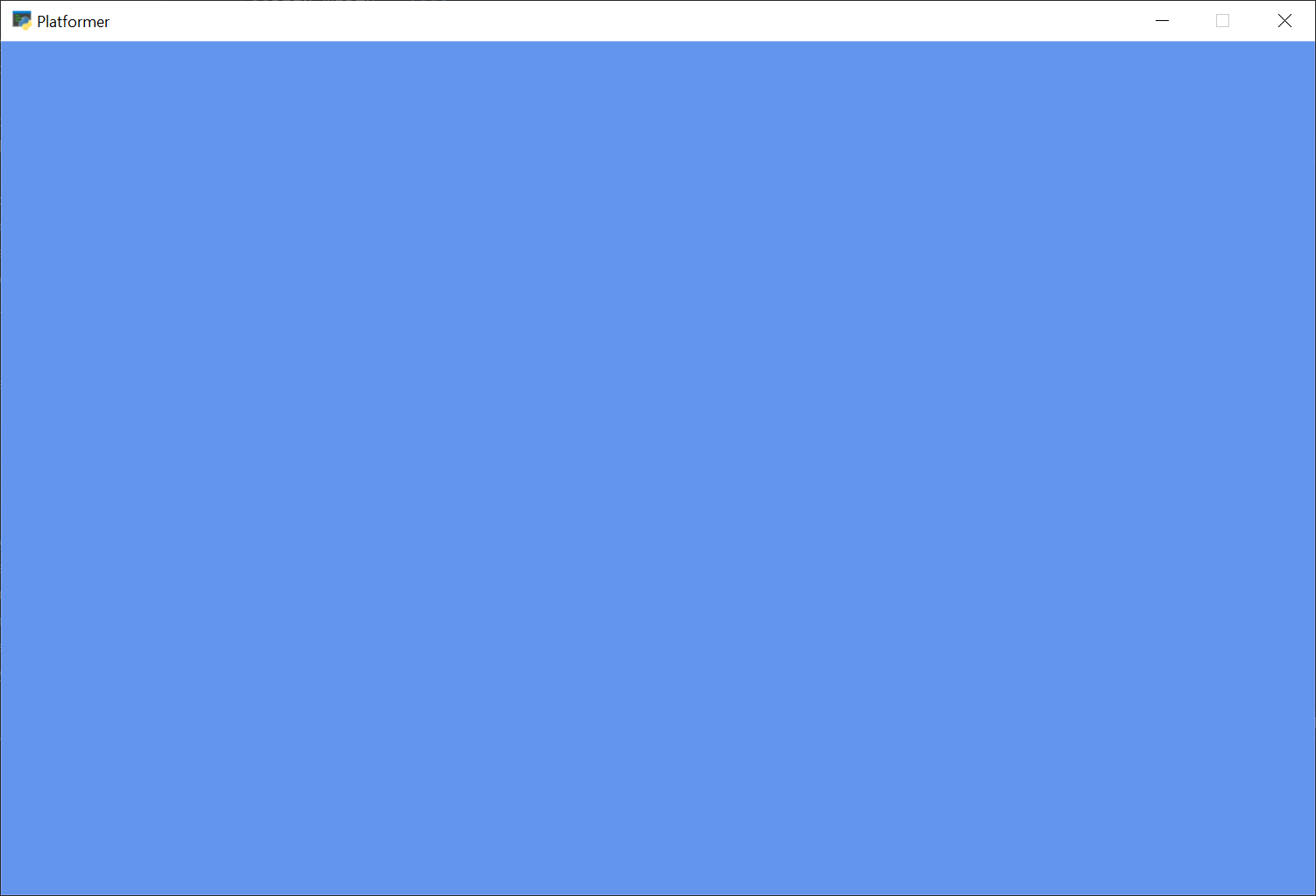## Step 2: Simple Shader Experiment

How do we know our shader is really working? As it is just straight copying everything across, it is hard to tell.

We can modify our shader to get the current texture color and store it in the variable `inColor`. A color has four components, red-green-blue and alpha. If the alpha is above zero, we can output a red color. If the alpha is zero, we output a blue color.

Note

Colors in OpenGL are specified in RGB or RGBA format. But instead of numbers going from 0-255, each component is a floating point number from 0.0 to 1.0.

GLSL Program for Step 2
```void mainImage( out vec4 fragColor, in vec2 fragCoord )
{
vec2 normalizedFragCoord = fragCoord/iResolution.xy;
vec4 inColor = texture(iChannel0, normalizedCoord);
if (inColor.a > 0.0)
// Set to a red color
fragColor = vec4(1.0, 0.0, 0.0, 1.0);
else
// Set to a blue color
fragColor = vec4(0.0, 0.0, 1.0, 1.0);
}
```

Giving us a resulting image that looks like: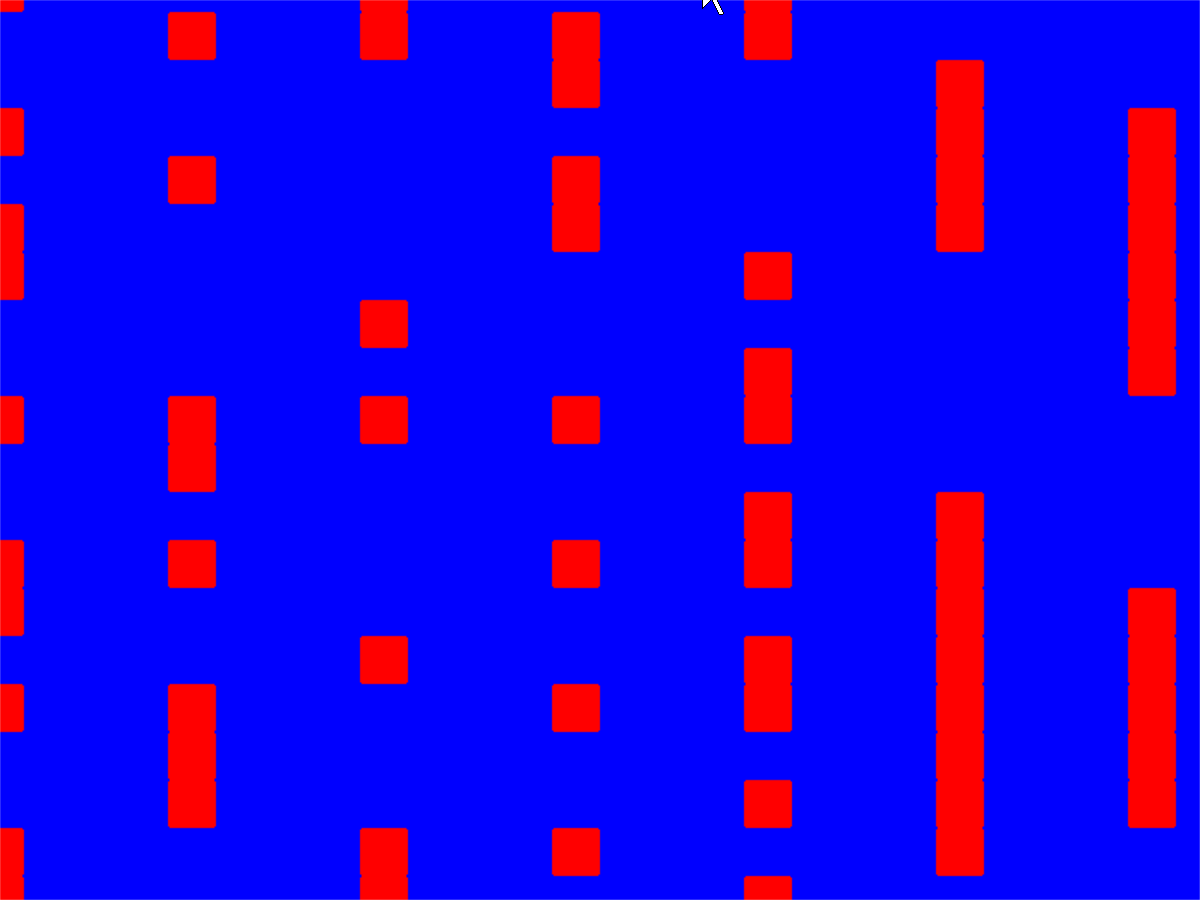## Step 3: Creating a Light

Our next step is to create a light. We’ll be fading between no light (black) and whatever we draw in Channel 1.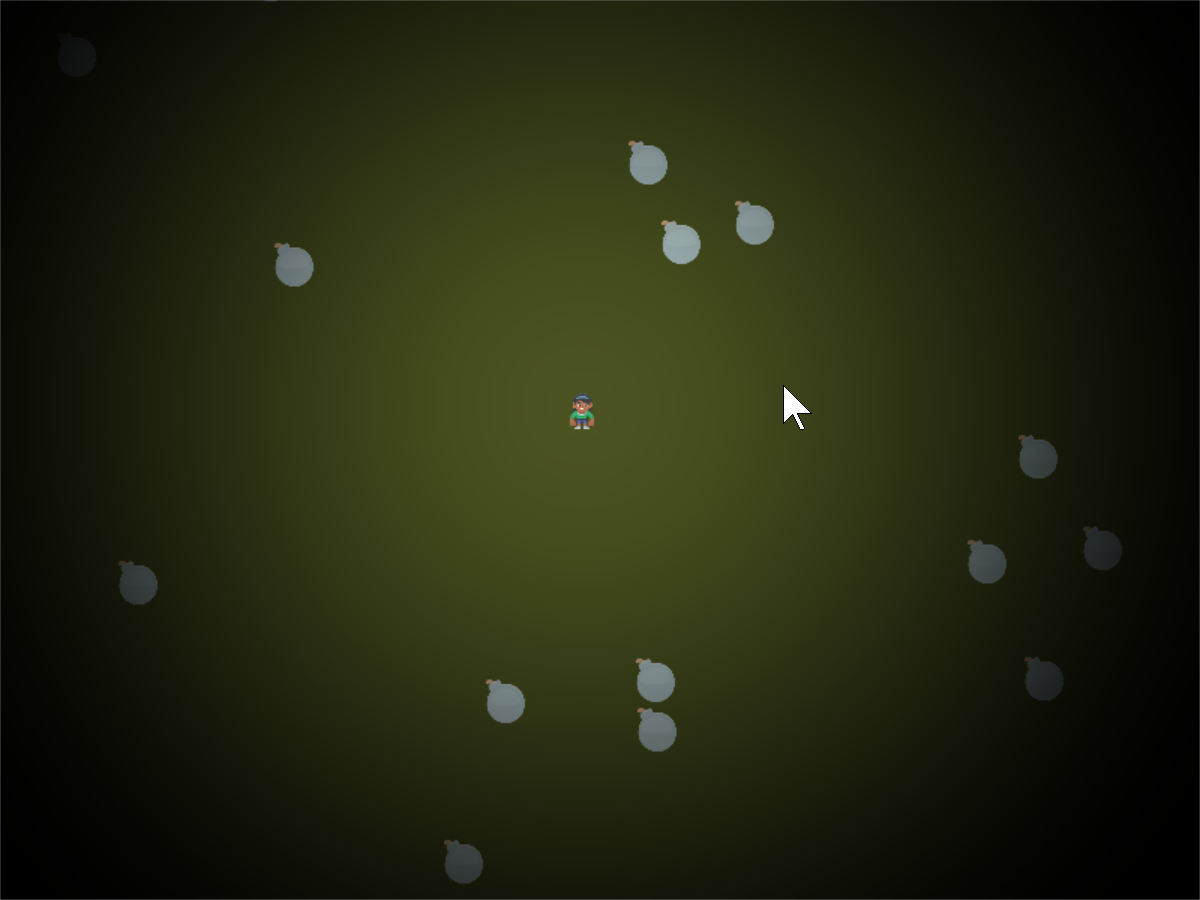In this step, we won’t worry about drawing the walls yet.

This step will require us to pass additional data into our shader. We’ll do this using uniforms. We will pass in where the light is, and the light size.

We first declare and use the variables in our shader program.

GLSL Program for Step 3
```// x, y position of the light
uniform vec2 lightPosition;
// Size of light in pixels
uniform float lightSize;
```

Next, we need to know how far away this point is from the light. We do that by subtracting this point from the light position. We can perform mathematical operations on vectors, so we just subtract. Then we use the build-in `length` function to get a floating point number of how long the length of this vector is.

GLSL Program for Step 3
```    // Distance in pixels to the light
float distanceToLight = length(lightPosition - fragCoord);
```

Next, we need to get the coordinate of the pixel we are calculating, but normalized. The coordinates will range from 0.0 to 1.0, with the left bottom of the window at (0,0), and the top right at (1,1). Normalized coordinates are used in shaders to make scaling up and down easy.

GLSL Program for Step 3
```    // Normalize the fragment coordinate from (0.0, 0.0) to (1.0, 1.0)
vec2 normalizedFragCoord = fragCoord/iResolution.xy;
```

Then we need to calculate how much light is falling on this coordinate. This number will also be normalized. A number of 0.0 will be in complete shadow, and 1.0 will be fully lit.

We will use the built-in `smoothstep` function that will take how large our light size is, and how far we are from the light. Then scale it from a number 0.0 to 1.0.

If we are 0.0 pixels from the light, we’ll get a 0.0 back. If we are halfway to the light we’ll get 0.5. If we are at the light’s edge, we’ll get 1.0. If we are beyond the light’s edge we’ll get 1.0.

Unfortunately this is backwards from what we want. We want 1.0 at the center, and 0.0 outside the light. So a simple subtraction from 1.0 will solve this issue.

GLSL Program for Step 3
```    // Start our mixing variable at 1.0
float lightAmount = 1.0;

// Find out how much light we have based on the distance to our light
lightAmount *= 1.0 - smoothstep(0.0, lightSize, distanceToLight);
```

Next, we are going to use the built-in `mix` function and the `lightAmount` variable to alternate between whatever is in channel 1, and a black shadow color.

GLSL Program for Step 3
```    // We'll alternate our display between black and whatever is in channel 1
vec4 blackColor = vec4(0.0, 0.0, 0.0, 1.0);

// Our fragment color will be somewhere between black and channel 1
// dependent on the value of b.
fragColor = mix(blackColor, texture(iChannel1, normalizedFragCoord), lightAmount);
```

Finally we’ll go back to the Python program and update our `on_draw` method to:

• Draw the bombs into channel 1.

• Send the player position and the size of the light using the uniform.

• Draw the player character on the window.

```    def on_draw(self):
# Select the channel 0 frame buffer to draw on
self.channel0.use()
# Draw the walls
self.wall_list.draw()

self.channel1.use()
# Draw the bombs
self.bomb_list.draw()

# Select this window to draw on
self.use()
# Clear to background color
self.clear()
# Run the shader and render to the window
# Draw the player
self.player_list.draw()
```

Note

If you set a uniform variable using `program`, that variable has to exist in the glsl program, and be used or you’ll get an error. The glsl compiler will automatically drop unused variables, causing a confusing error when the program says a variable is missing even if you’ve declared it.

• Step 3 Python ← Full listing of where we are right now with the Python program

• Step 3 GLSL ← Full listing of where we are right now with the GLSL program

• step_03.py Diff ← What we changed to get here

## Step 4: Make the Walls Shadowed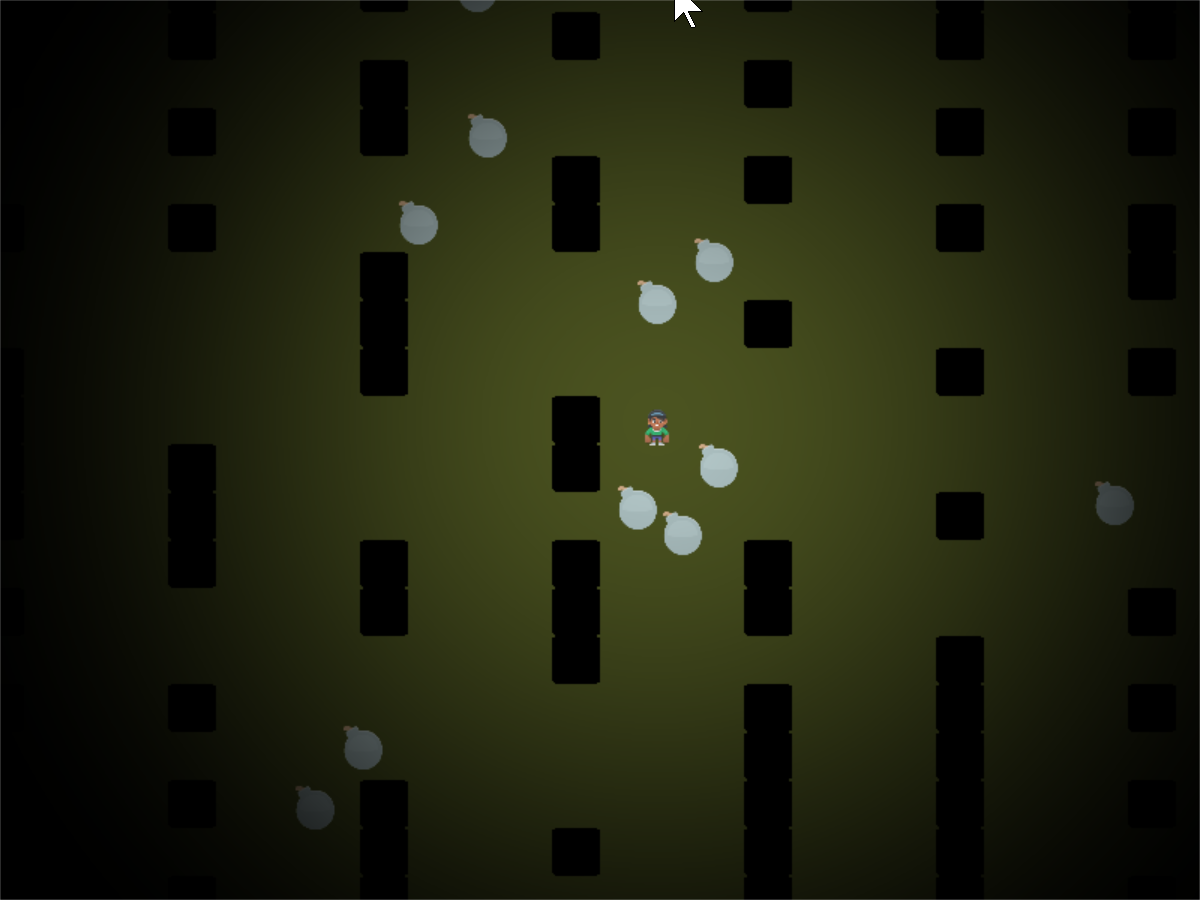In addition to the light, we want the walls to show up in shadow for this step. We don’t need to change our Python program at all for this, just the GLSL program.

First, we’ll add to our GLSL program a `terrain` function. This will sample channel 0. If the pixel there has an alpha of 0.1 or greater (a barrier to our light), we’ll use the `step` function and get 1.0. Otherwise we’ll get 0.0. Then, since we want this reversed, (0.0 for barriers, 1.0 for no barrier) we’ll subtract from 1.0:

GLSL Program for Step 4
```float terrain(vec2 samplePoint)
{
float samplePointAlpha = texture(iChannel0, samplePoint).a;
float sampleStepped = step(0.1, samplePointAlpha);
float returnValue = 1.0 - sampleStepped;

return returnValue;
}
```

Next, we’ll factor in this barrier to our light. So our light amount will be a combination of the distance from the light, and if there’s a barrier object on this pixel.

GLSL Program for Step 4
```    // Start our mixing variable at 1.0
float lightAmount = 1.0;

// Find out how much light we have based on the distance to our light
lightAmount *= 1.0 - smoothstep(0.0, lightSize, distanceToLight);
```

## Step 5: Cast the Shadows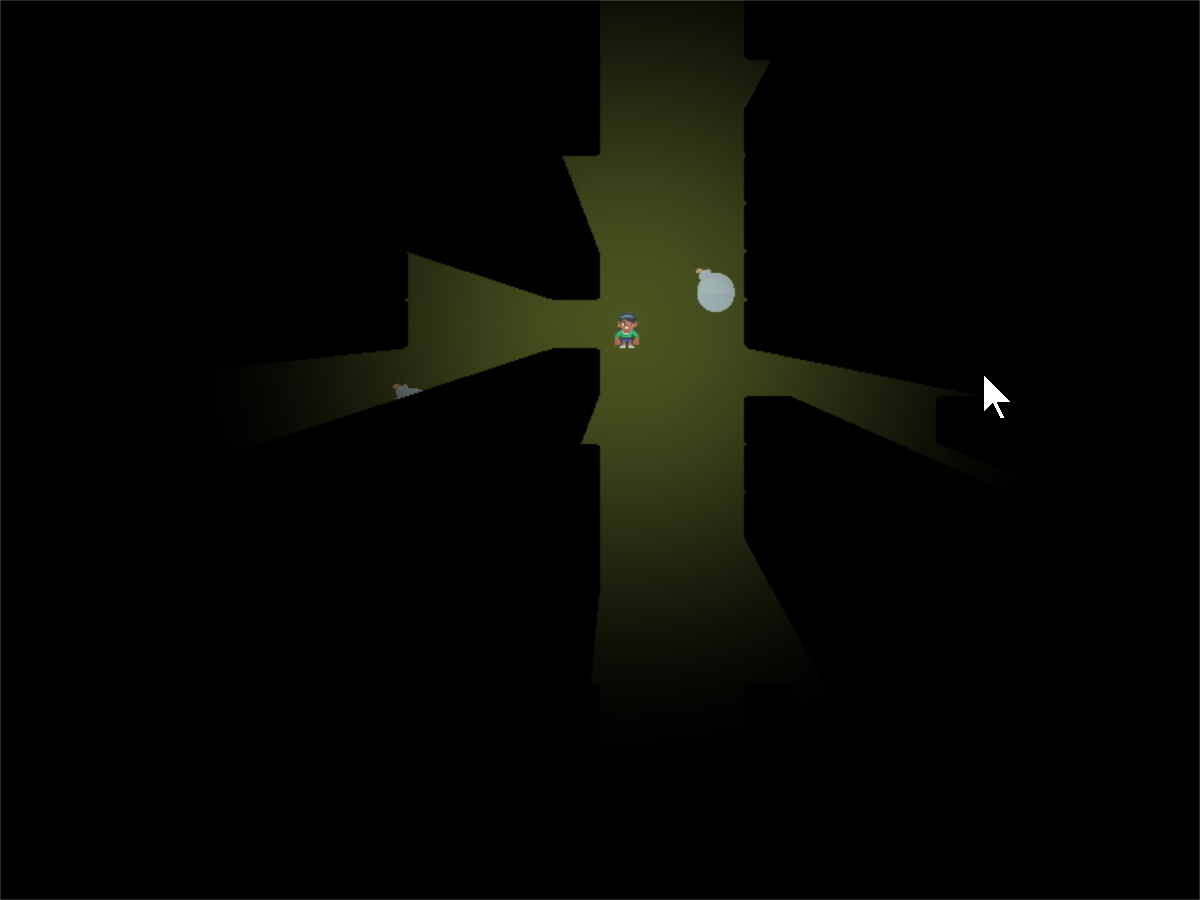Now it is time to cast the shadows.

This involves a lot of “sampling”. We start at our current point and draw a line to where the light is. We will sample “N” times along that line. If we spot a barrier, our coordinate must be in shadow.How many times do we sample? If we don’t sample enough times, we miss barriers and end up with weird shadows. This first image is if we only sample twice. Once where we are, and once in the middle: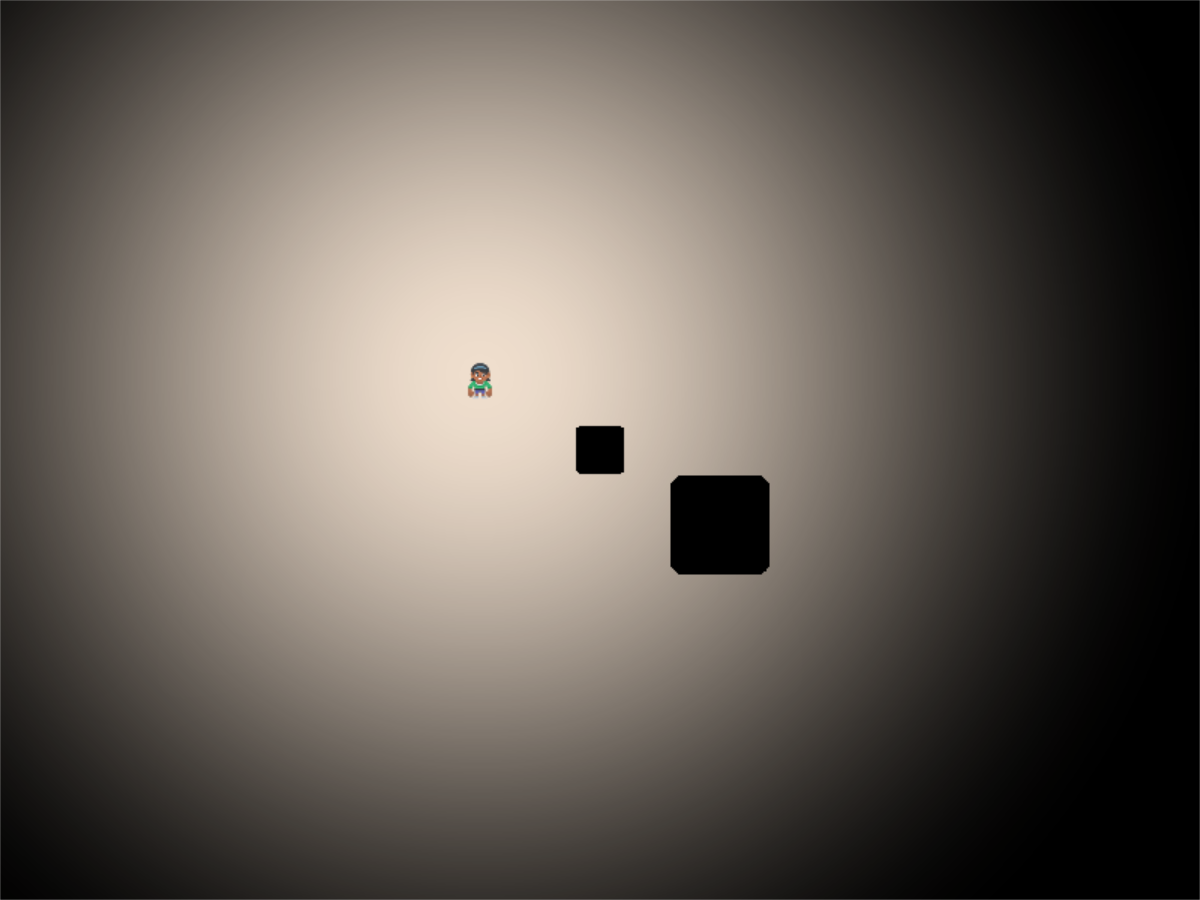If N is three, we end up with three copies of the shadow: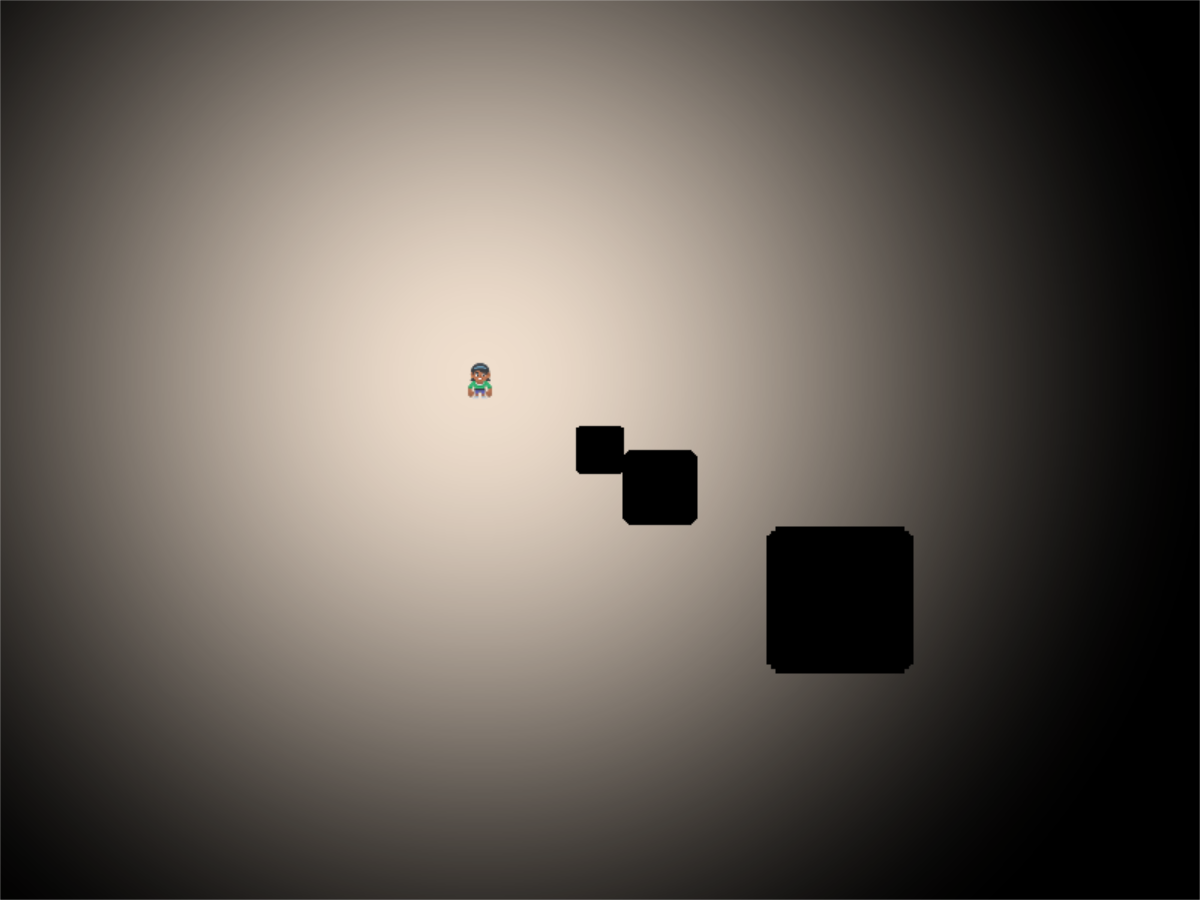With an N of 10: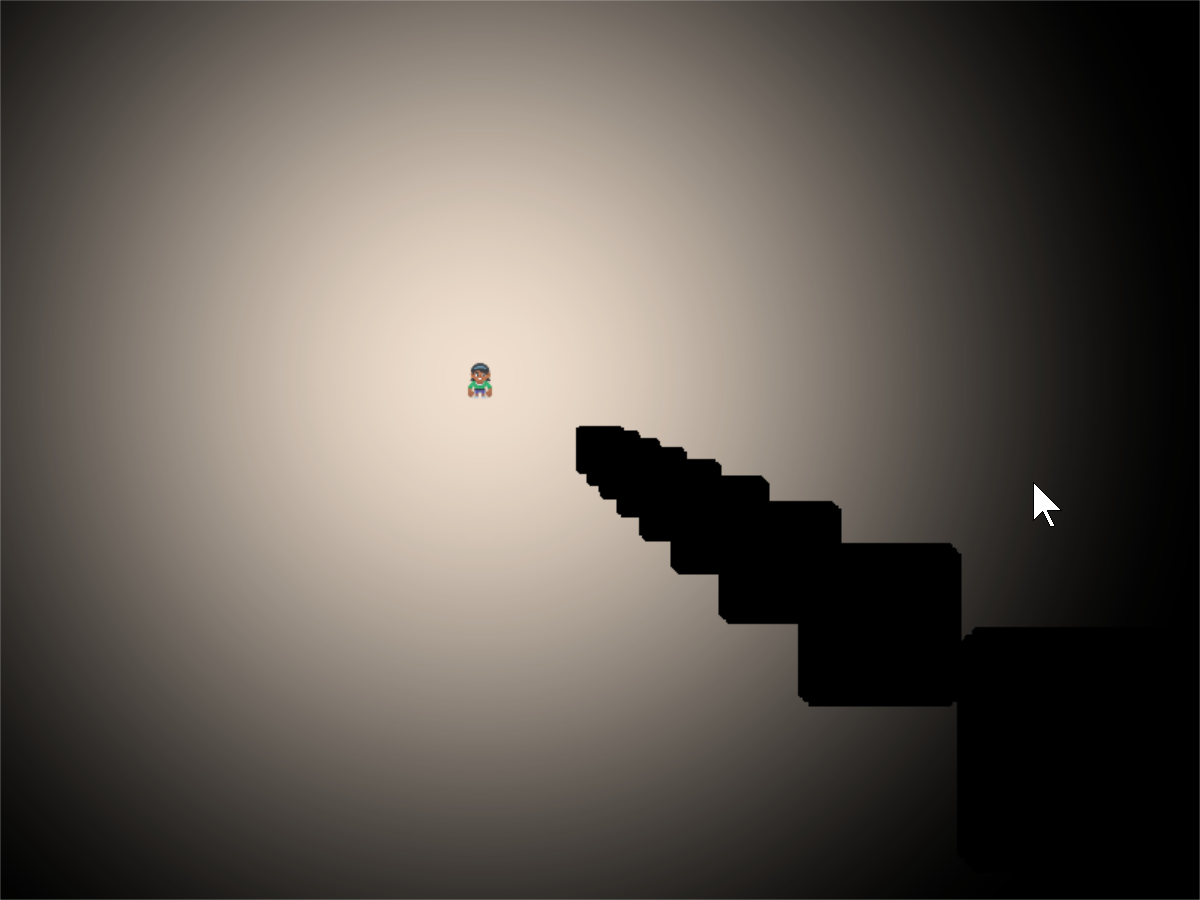We can use an N of 500 to get a good quality shadow. We might need more if your barriers are small, and the light range is large.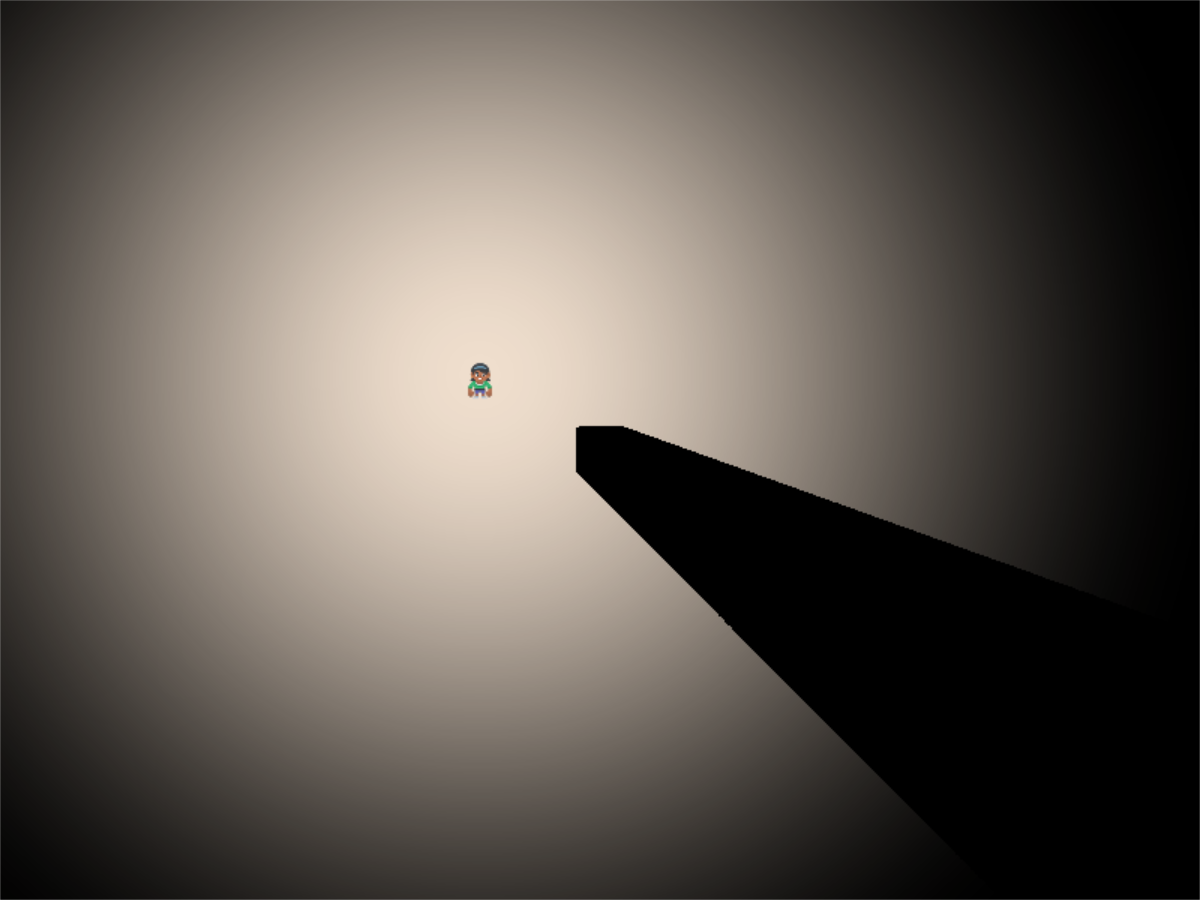Keep in mind there is a speed trade-off. With 800x600 pixels, we have 480,000 pixels to calculate. If each of those pixels has a loop that does 500 samples, we are sampling 480,000x500 = 240,000 sample per frame, or 14.4 million samples per second, still very do-able with modern graphics cards.

But what if you scale up? A 4k monitor would need 247 billion samples per second! There are optimizations that would be done, such as exiting out of the `for` loop once we are in shadow, and not calculating for points beyond the light’s range. We aren’t covering that here, but even with 2D, it will be important to understand what the shader is doing to keep reasonable performance.

## Step 6: Soft Shadows and Wall Drawing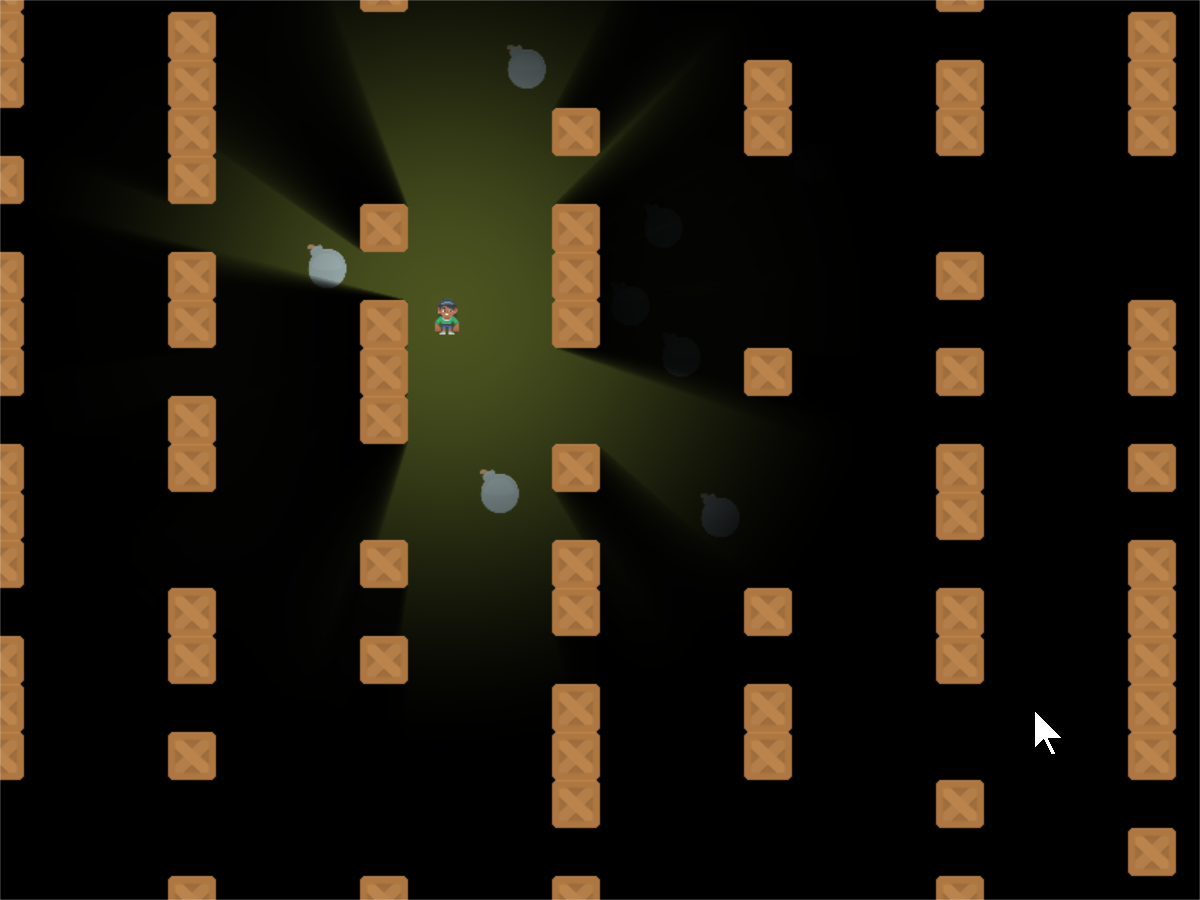With one more line of code, we can soften up the shadows so they don’t have such a “hard” edge to them.

To do this, modify the `terrain` function in our GLSL program. Rather than return 0.0 or 1.0, we’ll return 0.0 or 0.98. This allows edges to only partially block the light.

GLSL Program for Step 6
```float terrain(vec2 samplePoint)
{
float samplePointAlpha = texture(iChannel0, samplePoint).a;
float sampleStepped = step(0.1, samplePointAlpha);
float returnValue = 1.0 - sampleStepped;

// The closer the first number is to 1.0, the softer the shadows.
returnValue = mix(0.98, 1.0, returnValue);
```

And then we can go ahead and draw the barriers back on the screen so we can see what is casting the shadows.

Step 6, Draw the Barriers
```    def on_draw(self):
# Select the channel 0 frame buffer to draw on
self.channel0.use()
# Draw the walls
self.wall_list.draw()

self.channel1.use()
# Draw the bombs
self.bomb_list.draw()

# Select this window to draw on
self.use()
# Clear to background color
self.clear()
# Run the shader and render to the window

# Draw the walls
self.wall_list.draw()

# Draw the player
self.player_list.draw()
```
• Step 6 Python ← Full listing of where we are right now with the Python program

• Step 6 GLSL ← Full listing of where we are right now with the GLSL program

• step_06.glsl Diff ← What we changed to get here

## Bibliography

Before I wrote this tutorial I did not know how these shadows were made. I found the sample code Simple 2d Ray-Cast Shadow by jt which allowed me to very slowly figure out how to cast shadows.